Miscellaneous Formulas

The root mean squared standard deviation of a clusteris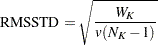The R-square statistic for a given level of the hierarchy is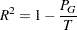The squared semipartial correlation for joining clustersandis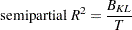The bimodality coefficient is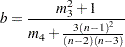whereis skewness andis kurtosis. Values of b greater than 0.555 (the value for a uniform population) can indicate bimodal or multimodal marginal distributions. The maximum of 1.0 (obtained for the Bernoulli distribution) is obtained for a population with only two distinct values. Very heavy-tailed distributions have small values of b regardless of the number of modes.

Formulas for the cubic-clustering criterion and approximate expected R square are given in Sarle (1983).

The pseudo F statistic for a given level is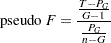The pseudo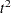statistic for joiningandis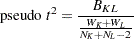The pseudo F andstatistics can be useful indicators of the number of clusters, but they are not distributed as F andrandom variables. If the data are independently sampled from a multivariate normal distribution with a scalar covariance matrix and if the clustering method allocates observations to clusters randomly (which no clustering method actually does), then the pseudo F statistic is distributed as an F random variable withand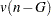degrees of freedom. Under the same assumptions, the pseudostatistic is distributed as an F random variable with v anddegrees of freedom. The pseudostatistic differs computationally from Hotelling’sin that the latter uses a general symmetric covariance matrix instead of a scalar covariance matrix. The pseudo F statistic was suggested by Calinski and Harabasz (1974). The pseudostatistic is related to the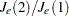statistic of Duda and Hart (1973) by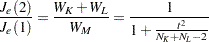See Milligan and Cooper (1985) and Cooper and Milligan (1988) regarding the performance of these statistics in estimating the number of population clusters. Conservative tests for the number of clusters using the pseudo F andstatistics can be obtained by the Bonferroni approach (Hawkins, Muller, and ten Krooden; 1982, pp. 337–340).